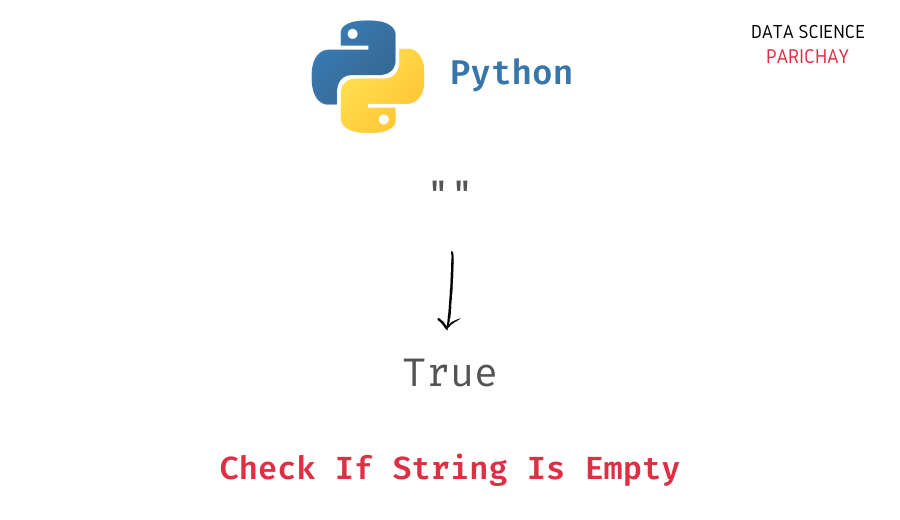# Python – Check If String Is Empty – With Examples

In this tutorial, we will look at how to check if a string is empty or not in Python with the help of some examples.

A string is empty if it does not contain any characters. You can use the following methods to check if a string is empty or not in Python.

• Comparing the string with an empty string.
• Using the `len()` function.
• Using the string in a boolean context.

Let’s now take a look at each of the above methods with the help of some examples.

You can also check if a string is empty or not by comparing it with an empty string, `""` using the `==` operator. Let’s look at an example.

```# create two strings - s1 empty and s2 non-empty
s1 = ""
s2 = "cat"
# check if string is empty
print(s1 == "")
print(s2 == "")```

Output:

```True
False```

We get `True` as the output for the string `s1` as it is empty and `False` for the string `s2` because it’s not empty (it contains some characters).

The length of an empty string is zero.

You can use the Python `len()` function to calculate the length of the string and then compare it with zero to check if it is empty or not. Here’s an example –

📚 Data Science Programs By Skill Level

Introductory

Intermediate ⭐⭐⭐

🔎 Find Data Science Programs 👨‍💻 111,889 already enrolled

Disclaimer: Data Science Parichay is reader supported. When you purchase a course through a link on this site, we may earn a small commission at no additional cost to you. Earned commissions help support this website and its team of writers.

```# create two strings - s1 empty and s2 non-empty
s1 = ""
s2 = "cat"
# check if string is empty
print(len(s1) == 0)
print(len(s2) == 0)```

Output:

```True
False```

We get the same results as above.

If you use a string in a boolean context, it will evaluate to `True` if it has any characters and it will evaluate to `False` if it is empty. Thus, you can use the expression `not s` to check if the string `s` is empty or not.

Here’s an example.

```# create two strings - s1 empty and s2 non-empty
s1 = ""
s2 = "cat"
# check if string is empty
print(not s1)
print(not s2)```

Output:

```True
False```

We get the same result as above. `True` for the string `s1` as it’s empty and `False` for the string `s2` as it’s not empty (`s2` has three characters).

In this tutorial, we looked at three methods to check if a string is empty or not. Use the method that you are the most comfortable with.

You might also be interested in –

•